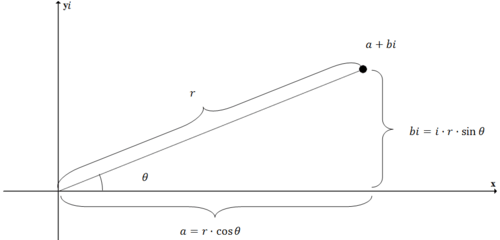# 5.3.6: Trigonometric Form of Complex Numbers

•• Contributed by CK12
• CK12

x plus y times i equals r times the sum of cosine theta and i times sine theta.

You already know how to represent complex numbers in the complex plane using rectangular coordinates and you already know how to multiply and divide complex numbers.  Representing these points and performing these operations using trigonometric polar form will make your computations more efficient.

What are the two ways to multiply the following complex numbers?

$$(1+\sqrt{3}i)(\sqrt{2}−\sqrt{2}i)$$

### Trigonometric Polar Form of Complex Numbers

Any point represented in the complex plane as $$a+bi$$ can be represented in polar form just like any point in the rectangular coordinate system.  Trigonometric polar form of a complex number describes the location of a point on the complex plane using the angle and the radius of the point. You will use the distance from the point to the origin as r and the angle that the point makes as $$\theta$$.Figure $$\PageIndex{1}$$

As you can see, the point a+bi can also be represented as $$r\cdot \cos \theta +i\cdot r\cdot \sin \theta$$.  The trigonometric polar form can be abbreviated by factoring out the r and noting the first letters:

$$r(\cos \theta +i\cdot \sin \theta )\rightarrow r\cdot \; cis \; \theta$$

The abbreviation $$r\cdot \; cis \; \theta$$ is read as “$$r$$ kiss theta.”  It allows you to represent a point as a radius and an angle.

Take the following complex number in rectangular form

$$1−\sqrt{3}i$$

To convert the following complex number from rectangular form to trigonometric polar form, find the radius using the absolute value of the number.

$$r^2 =1^2+(−\sqrt{3})^2\rightarrow r=2$$

The angle can be found with basic trig and the knowledge that the opposite side is always the imaginary component and the adjacent side is always the real component.

$$\tan \theta =−\dfrac{\sqrt{3}}{1} \rightarrow \theta =60^{\circ}$$

Thus the trigonometric form is $$2 \; cis \; 60^{\circ}$$.

One great benefit of the cis form is that it makes multiplying and dividing complex numbers extremely easy.  For example:

Let: $$z_1 =r_1 \cdot \; cis \; \theta_1 ,z_2 =r_2 \cdot \; cis \; \theta_2$$ with $$r_2 \neq 0$$.

Then:

\begin{aligned} z_1 \cdot z_2 &=r_1 \cdot r_2 \cdot \; cis \; (\theta_1 +\theta_2) \\ z_1 \div z_2 &=\dfrac{r_1 }{r_2} \cdot \; cis \;(\theta_1 −\theta_2) \end{aligned}

For basic problems, the amount of work required to compute products and quotients for complex numbers given in either form is roughly equivalent.  For more challenging questions, trigonometric polar form becomes significantly advantageous.

Example $$\PageIndex{1}$$

Earlier, you were asked how to multiply the complex numbers $$(1+\sqrt{3}i)(\sqrt{2}−\sqrt{2}i)$$.

Solution

In rectangular coordinates:

$$(1+\sqrt{3}i)(\sqrt{2}−\sqrt{2}i)=\sqrt{2}−\sqrt{2}i+\sqrt{6} i+\sqrt{6}$$

In trigonometric polar coordinates, $$1+\sqrt{3}i=2 \; cis \; 60^{\circ}$$ and $$\sqrt{2}−\sqrt{2}i=2 \; cis \;−45^{\circ}$$.  Therefore:

$$(1+\sqrt{3}i)(\sqrt{2}−\sqrt{2}i)=2 \; cis \; 60^{\circ} \cdot 2 \; cis \;−45^{\circ} =4 \; cis \; 105^{\circ}$$

Example $$\PageIndex{2}$$

Convert the following complex number from trigonometric polar form to rectangular form.

$$4 \; cis \;(3\dfrac{\pi}{4})$$

Solution

$$4 \; cis \; \left(3\dfrac{\pi}{4}\right)=4\left(\cos \left(\dfrac{3 \pi}{4}\right)+i\cdot \sin \left(\dfrac{3 \pi}{4}\right)\right)=4\left(−\dfrac{\sqrt{2}}{2}+\dfrac{\sqrt{2}}{2}i\right)=−2\sqrt{2}+2\sqrt{2}i$$

Example $$\PageIndex{3}$$

Divide the following complex numbers.

$$\dfrac{4 \; cis \; 32^{\circ} }{2 \; cis \; 2^{\circ}}$$

Solution

$$\dfrac{4 \; cis \; 32^{\circ} }{2 \; cis \; 2^{\circ} }=42 \; cis \; (32^{\circ} −2^{\circ} )=2 \; cis \; (30^{\circ} )$$

Example $$\PageIndex{4}$$

Translate the following complex number from rectangular form into trigonometric polar form:

8

Solution

$$8=8 \; cis \; 0^{\circ}$$

Note that this has no complex part and therefore has no angle.

Example $$\PageIndex{5}$$

Multiply the following complex numbers in trigonometric polar form.

$$4 \; cis \; 34^{\circ} \cdot 5 \; cis \; 16^{\circ} \cdot \dfrac{1}{2} \; cis \; 100^{\circ}$$

Solution

\begin{aligned} 4 \; cis \; 34^{\circ} \cdot 5 \; cis \; 16^{\circ} \cdot \dfrac{1}{2} \; cis \; 100^{\circ} \\ &=4\cdot 5\cdot 12\cdot \; cis \; (34^{\circ} +16^{\circ} +100^{\circ} ) \\ &=10 \; cis \; 150 \end{aligned}

Note how much easier it is to do products and quotients in trigonometric polar form.

## Review

Translate the following complex numbers from trigonometric polar form to rectangular form.

1. $$5 \; cis \; 270^{\circ}$$

2. $$2 \; cis \; 30^{\circ}$$

3. $$−4 \; cis \; \dfrac{\pi}{4}$$

4. $$6 \; cis \; \dfrac{\pi}{3}$$

5. $$2 \; cis \; \dfrac{5\pi}{2}$$

Translate the following complex numbers from rectangular form into trigonometric polar form.

6. $$2−i$$

7. $$5+12i$$

8. $$6i+8$$

9. $$i$$

Complete the following calculations and simplify.

10. $$2 \; cis \; 22^{\circ} \cdot \dfrac{1}{5} \; cis \; 15^{\circ} \cdot 3 \; cis \; 95^{\circ}$$

11. $$9 \; cis \; 98^{\circ} \div 3 \; cis \; 12^{\circ}$$

12. $$15 \; cis \; \dfrac{\pi}{4}\cdot 2 \; cis \; \dfrac{\pi}{6}$$

13. $$−2 \; cis \; \dfrac{2\pi}{3}\div 15 \; cis \; \dfrac{7 \pi}{6}$$

Let $$z_1 =r_1 \cdot \; cis \; \theta_1$$ and $$z_2 =r_2 \cdot \; cis \; \theta_2$$ with r_2 \neq 0\).

14.  Use the trigonometric sum and difference identities to prove that $$z_1 \cdot z_2 =r_1 \cdot r_2 \cdot \; cis \; (\theta_1 +\theta_2)$$.

15.  Use the trigonometric sum and difference identities to prove that $$z_1 \div z_2 =r_1 r_2 \cdot \; cis \; (\theta_1 −\theta_2)$$.

To see the Review answers, open this PDF file and look for section 11.3.

## Vocabulary

Term Definition
$$r\; cis \;\theta$$ $$r\; cis \;\theta$$ is shorthand for the expression $$r\cos \theta +ri\sin \theta$$.
complex plane The complex plane is the graphical representation of the set of all complex numbers.
polar form The polar form of a point or a curve is given in terms of r and $$\theta$$ and is graphed on the polar plane.
rectangular form The rectangular form of a point or a curve is given in terms of x and y and is graphed on the Cartesian plane.
trigonometric form To write a complex number in trigonometric form means to write it in the form $$r\cos \theta +ri\sin \theta$$. $$r\; cis \;\theta$$ is shorthand for this expression.
trigonometric polar form To write a complex number in trigonometric form means to write it in the form $$r\cos \theta +ri\sin \theta$$. $$r\; cis \;\theta$$ is shorthand for this expression.